## Thursday, November 30, 2017

### Visualizing the rate of change in a discrete character through time

A friend & colleague asked me about trying to track reconstructed changes in a discrete character across the tree with the idea of testing the hypothesis that changes are distributed heterogeneously through time.

The following is one relatively simple idea that is to conduct stochastic mapping & then visualize the mean number of sampled changes by time segment across the tree. This is not precisely the same as fitting a model that is heterogeneous in rate across time; however it might nonetheless give a reasonable idea of what is going on.

``````library(phytools)
tree
``````
``````##
## Phylogenetic tree with 200 tips and 199 internal nodes.
##
## Tip labels:
##  t8, t24, t25, t60, t61, t21, ...
##
## Rooted; includes branch lengths.
``````
``````x
``````
``````##   t8  t24  t25  t60  t61  t21  t22  t41 t162 t163  t76  t17 t137 t138 t100
##    b    b    b    b    b    b    b    b    b    b    b    b    b    b    b
##  t67  t50 t179 t180  t69 t127 t128 t132 t133  t82   t2  t34  t35  t44  t45
##    c    b    b    b    c    c    c    b    b    b    b    b    b    b    b
##  t62  t63  t47 t146 t147  t68  t93  t94  t74 t197 t198 t199 t200  t87  t71
##    a    a    b    a    a    b    b    a    b    b    b    c    c    c    c
##  t38 t154 t155   t7  t27  t28   t9  t10  t11 t152 t153 t149 t125 t135 t136
##    c    c    c    b    b    b    a    b    b    b    b    b    b    b    b
##  t54  t14  t15 t181 t182  t12  t13 t108 t161 t175 t176  t42  t37   t6  t78
##    b    b    b    b    b    b    c    c    c    c    c    c    c    b    b
##  t79  t18  t30  t31  t88  t89  t53  t55 t114 t115  t49 t109 t110  t20 t118
##    b    b    b    b    b    b    b    b    b    b    b    b    b    b    b
## t119 t104 t171 t172 t148  t26  t65  t66   t1  t91  t92  t70  t23 t195 t196
##    b    b    b    b    b    b    a    b    b    b    b    b    b    b    b
## t158  t64 t173 t174 t166 t167  t29 t111 t112 t105  t19 t116 t117  t83  t52
##    b    b    b    b    c    c    b    b    b    b    b    b    b    b    b
##  t39  t40 t120 t121 t193 t194 t150 t151  t98  t99  t80  t81  t46  t58  t59
##    b    b    b    b    b    b    a    a    a    a    a    a    c    a    a
## t130 t131 t129 t169 t170 t168 t159 t160 t191 t192   t5 t102 t103  t77  t95
##    a    a    a    a    a    a    a    a    a    a    a    b    b    b    b
##  t96  t43 t144 t145  t90  t97 t124 t156 t157 t139  t51 t189 t190 t101  t33
##    b    b    b    a    b    b    b    b    b    b    c    c    c    c    c
##  t86 t185 t186 t113 t183 t184 t164 t165 t106 t107  t75  t32 t140 t141 t134
##    c    c    c    c    c    c    c    c    c    c    c    c    c    c    c
## t122 t123 t142 t143 t126 t187 t188  t84  t85  t16  t72  t73 t177 t178   t3
##    c    c    c    c    c    c    c    b    b    b    b    a    b    b    b
##   t4  t56  t57  t48  t36
##    b    c    c    c    c
## Levels: a b c
``````
``````trees<-make.simmap(tree,x,model="ER",nsim=100)
``````
``````## make.simmap is sampling character histories conditioned on the transition matrix
##
## Q =
##              a            b            c
## a -0.004767771  0.002383886  0.002383886
## b  0.002383886 -0.004767771  0.002383886
## c  0.002383886  0.002383886 -0.004767771
## (estimated using likelihood);
## and (mean) root node prior probabilities
## pi =
##         a         b         c
## 0.3333333 0.3333333 0.3333333
``````
``````## Done.
``````
``````trees
``````
``````## 100 phylogenetic trees with mapped discrete characters
``````

First, phytools has a function for plotting the posterior distribution of changes directly on the tree. It also returns to the environment the positions of all these changes on the tree.

``````library(RColorBrewer)
colors<-setNames(brewer.pal(3,"Set1"),letters[1:3])
plotTree(tree,ftype="off",lwd=1)
par(fg="transparent")
tiplabels(pie=to.matrix(x,letters[1:3]),piecol=colors,cex=0.2)
par(fg="black")
changes<-sapply(trees,markChanges,
colors=sapply(colors,make.transparent,alpha=0.3))
``````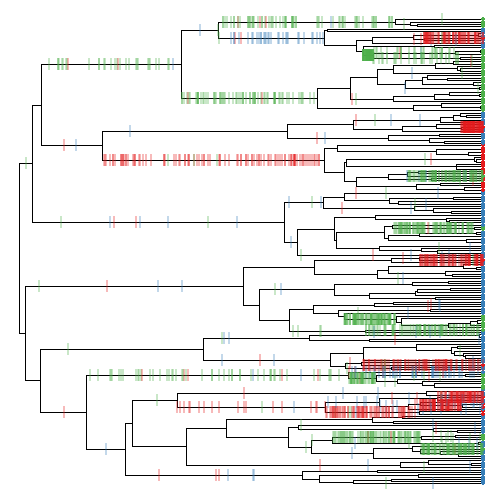Note that these are not marked changes from one history, but from a sample of 100 such histories on the tree.

The object returned by this set of calls to `markChanges` looks as follows:

``````head(changes,n=2)
``````
``````## []
##             x         y
## b->c 92.39156  16.00000
## b->c 79.08969  20.75000
## b->a 62.74820  33.65625
## a->b 95.08825  33.00000
## a->b 97.62994  36.00000
## a->b 97.40935  37.00000
## b->c 75.98806  46.28125
## b->a 78.73534  52.00000
## b->c 81.91755  67.00000
## b->c 74.75532  71.71875
## b->a 88.76125  97.00000
## b->c 82.54870 110.50000
## b->c 62.94025 121.56250
## c->b 64.89966 121.56250
## b->a 31.11766 139.70312
## a->c 90.50957 133.00000
## b->a 96.94503 154.00000
## b->c 25.29105 181.05078
## c->a 47.65342 192.20312
## a->b 60.05642 192.20312
## b->c 79.20952 183.50000
## b->c 81.67096 185.75000
## b->a 94.56798 192.00000
##
## []
##             x         y
## b->c 99.36067  16.00000
## b->c 75.13884  20.75000
## b->a 69.79316  31.50000
## b->a 87.57828  34.50000
## b->a 98.22282  38.00000
## b->c 26.20428  47.64062
## c->b 85.50603  49.00000
## b->a 75.34404  52.00000
## b->c 91.32295  67.00000
## b->c 76.94021  71.71875
## b->a 99.43863  97.00000
## b->c 86.20844 110.50000
## b->a 22.31200 139.70312
## a->c 97.90611 133.00000
## b->a 96.23615 154.00000
## b->c 36.15047 166.43750
## b->c 74.66465 184.62500
## b->a 87.48430 192.00000
## b->c 52.34096 199.12500
``````

Now let's go about trying to compute the average number of changes for each of 20 (say) temporal segments from the root of the tree to the present.

``````h<-max(nodeHeights(tree))
b<-20
segs<-cbind(seq(0,h-h/b,h/b),
seq(1/b*h,h,h/b))
segs
``````
``````##       [,1] [,2]
##  [1,]    0    5
##  [2,]    5   10
##  [3,]   10   15
##  [4,]   15   20
##  [5,]   20   25
##  [6,]   25   30
##  [7,]   30   35
##  [8,]   35   40
##  [9,]   40   45
## [10,]   45   50
## [11,]   50   55
## [12,]   55   60
## [13,]   60   65
## [14,]   65   70
## [15,]   70   75
## [16,]   75   80
## [17,]   80   85
## [18,]   85   90
## [19,]   90   95
## [20,]   95  100
``````

These will be our segments. (The total tree length is 100.)

Now let's compute the mean number of sampled changes for each segment:

``````nchanges<-rep(0,b)
for(i in 1:length(changes)){
for(j in 1:nrow(changes[[i]])){
ind<-which((changes[[i]][j,1]>segs[,1])+
(changes[[i]][j,1]<=segs[,2])==2)
nchanges[ind]<-nchanges[ind]+1/length(changes)
}
}
plot(h-as.vector(t(segs)),rbind(nchanges,nchanges),type="l",lwd=2,
xlim=c(max(segs),min(segs)),
lend=0,xlab="time since the present",ylab="mean number of changes")
mar=par()\$mar,direction="leftwards",xlim=c(max(segs),min(segs)))
``````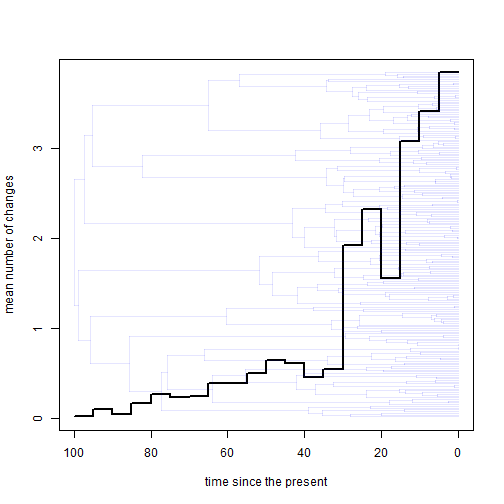Neat. Of course, under a constant process we expect the number of changes to increase towards the present due to the greater edge length of that part of the tree. To control for this we could also compute the total edge length of each segment. This is a bit tricky, but we can do it as follows:

``````LTT<-ltt(tree,plot=FALSE)
LTT<-cbind(LTT\$ltt[2:(length(LTT\$ltt)-1)],
LTT\$times[2:(length(LTT\$ltt)-1)],
LTT\$times[3:length(LTT\$ltt)])
ii<-1
edge.length<-rep(0,b)
for(i in 1:nrow(segs)){
done.seg<-FALSE
while(LTT[ii,2]<=segs[i,2]&&done.seg==FALSE){
edge.length[i]<-edge.length[i]+
LTT[ii,1]*(min(segs[i,2],LTT[ii,3])-
max(segs[i,1],LTT[ii,2]))
if(LTT[ii,3]>=segs[i,2]) done.seg<-TRUE
if(LTT[ii,3]<=segs[i,2]) ii<-if(ii<nrow(LTT)) ii+1 else ii
}
}
``````

Now let's plot the mean number of changes per segment, divided by the total edge length encompassed by the segment:

``````plot(h-as.vector(t(segs)),
rbind(nchanges/edge.length,nchanges/edge.length),type="l",lwd=2,
xlim=c(max(segs),min(segs)),
lend=0,xlab="time since the present",
ylab="mean number of changes / total edge length")
mar=par()\$mar,direction="leftwards",xlim=c(max(segs),min(segs)))
``````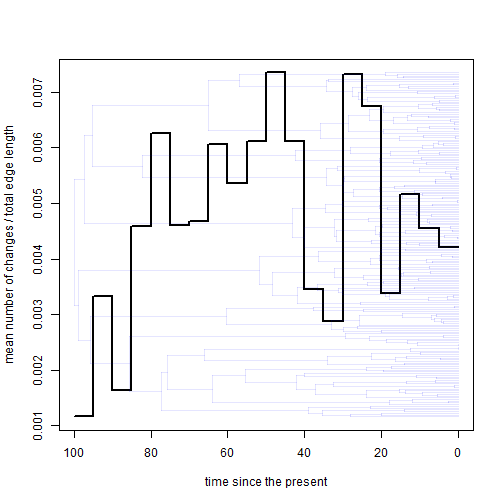Cool.

Now we can undertaken the same procedure, but this time with a different character, y:

``````trees<-make.simmap(tree,y,model="ER",nsim=100)
``````
``````## make.simmap is sampling character histories conditioned on the transition matrix
##
## Q =
##              a            b            c
## a -0.002287677  0.001143839  0.001143839
## b  0.001143839 -0.002287677  0.001143839
## c  0.001143839  0.001143839 -0.002287677
## (estimated using likelihood);
## and (mean) root node prior probabilities
## pi =
##         a         b         c
## 0.3333333 0.3333333 0.3333333
``````
``````## Done.
``````
``````plotTree(tree,ftype="off",lwd=1)
par(fg="transparent")
tiplabels(pie=to.matrix(x,letters[1:3]),piecol=colors,cex=0.2)
par(fg="black")
changes<-sapply(trees,markChanges,
colors=sapply(colors,make.transparent,alpha=0.3))
``````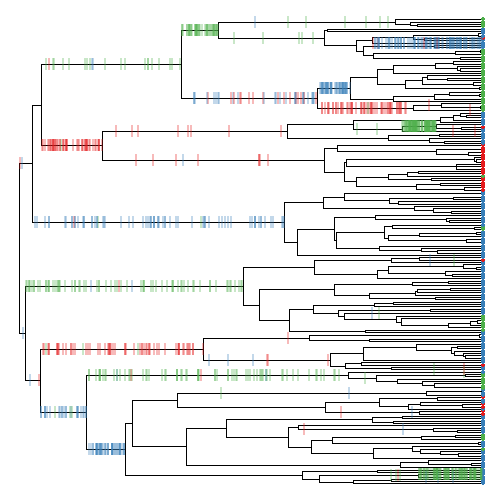``````h<-max(nodeHeights(tree))
b<-20
segs<-cbind(seq(0,h-h/b,h/b),
seq(1/b*h,h,h/b))
nchanges<-rep(0,b)
for(i in 1:length(changes)){
for(j in 1:nrow(changes[[i]])){
ind<-which((changes[[i]][j,1]>segs[,1])+
(changes[[i]][j,1]<=segs[,2])==2)
nchanges[ind]<-nchanges[ind]+1/length(changes)
}
}
LTT<-ltt(tree,plot=FALSE)
LTT<-cbind(LTT\$ltt[2:(length(LTT\$ltt)-1)],
LTT\$times[2:(length(LTT\$ltt)-1)],
LTT\$times[3:length(LTT\$ltt)])
ii<-1
edge.length<-rep(0,b)
for(i in 1:nrow(segs)){
done.seg<-FALSE
while(LTT[ii,2]<=segs[i,2]&&done.seg==FALSE){
edge.length[i]<-edge.length[i]+
LTT[ii,1]*(min(segs[i,2],LTT[ii,3])-
max(segs[i,1],LTT[ii,2]))
if(LTT[ii,3]>=segs[i,2]) done.seg<-TRUE
if(LTT[ii,3]<=segs[i,2]) ii<-if(ii<nrow(LTT)) ii+1 else ii
}
}
plot(h-as.vector(t(segs)),
rbind(nchanges/edge.length,nchanges/edge.length),type="l",lwd=2,
xlim=c(max(segs),min(segs)),
lend=0,xlab="time since the present",
ylab="mean number of changes / total edge length")
mar=par()\$mar,direction="leftwards",xlim=c(max(segs),min(segs)))
``````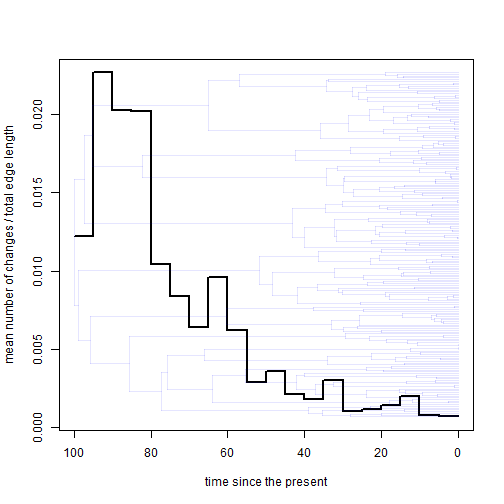This plot shows a greater concentration of changes (controlling for total edge length) towards the base of the tree. In fact, that is what I simulated by using an 'EB' transformation of the tree for simulation!

Data were simulated as follows:

``````tree<-pbtree(n=200,scale=100)
Q<-matrix(c(-0.004,0.002,0.002,
0.002,-0.004,0.002,
0.002,0.002,-0.004),3,3)
rownames(Q)<-colnames(Q)<-letters[1:3]
x<-as.factor(sim.history(tree,Q)\$states)
EB<-phytools:::ebTree(tree,-0.06)
EB\$edge.length<-EB\$edge.length/max(nodeHeights(EB))*100
y<-as.factor(sim.history(EB,10*Q)\$states)
``````